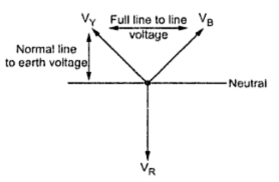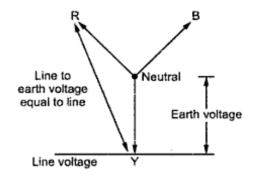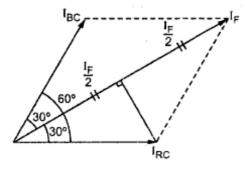### Underground or Isolated Neutral System

As the name indicates, the system neutral is not connected to the earth in this system. Thus the neutral is isolated from the earth. A simple isolated neutral system is shown in the Fig. 1(a).
There is always capacitive coupling between conductors and earth which causes capacitive currents to flow in the system. The line conductors have capacitance between one another and to earth. The capacitance between conductors is represented in delta while capacitance between conductor and earth is in star for 3 phases. As the grounding characteristics of the system are little affected by line characteristics., they can be neglected.Fig. 1
The capacitance to earth of each phase is uniformly distributed along its entire length and for all the calculations this capacitance is grouped to form a single capacitor connected between each phase and earth. These currents lead their respective voltage by 90o as shown in the phasor diagram. Fig. 1(b).
For a perfectly transposed line (symmetrically spaced) the capacitive currents IRC, IYC and IBC are equal in magnitude and displaced from each other by an angle of  120o. In balanced load condition with symmetrical spacing between conductors, the potential of neutral will be equal to that of earth as shown in the Fig. 2.Fig. 2  Potential of each phase to earth before fault
The charging currents IRC, IYC and IBC are balanced and their resultant is zero and no current flows to the earth. Now let us consider the earth fault on Y phase say at point P. The corresponding phase will be at earth potential while while the remaining healthy phases acquire line value from phase value as shown in the Fig. 3.Fig. 3
The capacitive current will not flow in this phase. The potential of neutral is not zero but it is shifted from earth potential position to position shown in the Fig. 3.
The circuit diagram and the corresponding phasor diagram during the fault is as shown in the Fig. 4.Fig. 4
Under fault condition, the capacitive currents are unbalanced and the fault current flows through the faulty line, into the fault and returns to the system via earth and through the earth capacitances  CR and CB. The current in Y line has two components one IRC and other IBC and the respective voltages driving these currents are VRY and VYB and the phase difference between currents and voltages is 90o due to capacitive nature of the impedance of the circuit. The vector sum of IRC and IBC gives fault current If.
Voltage to earth of R phase = √3 . VR
Voltage to earth of B phase = √3 . VB
We have,           IRC = VRY/XRC = (√3Vphase) / XC
Similarly we have,
IBC = VYB/XBC =  (√3Vphase) / XC
Both Vphase and XC are equal and the fault current is given by vector sum of and as shown in the Fig. 5.Fig. 5

From the diagram,
cos 30o = (If / 2)/IRC
If /2 = IRC . cos 30o
If /2 = IRC . (√3/2)
I= √3 . IRC
I= 3 . (√3Vphase / XC )
I= 3Vphase / XC
From the above equation, it can be seen that the current flowing through faulty phase is there times the normal line to neutral capacitive current flowing in each phase of the healthy system.
The following observations can be seen from the above analysis.
1. With an underground neutral system, if there is phase to earth fault then the voltages of the healthy phases with respect to earth rise from normal phase to neutral value towards full line value which may result in insulation breakdown.
2. The capacitive current in the remaining healthy phases increases to √3 times its normal value.
3. The capacitive current in the faulty phases is 3 times its normal value.
4. A capacitive current flows into the earth. If its magnitude is in excess of 4 to 5 amperes then it is sufficient to maintain an arc in the ionized path of the fault. This current can remain even after clearance of fault. This phenomenon of persistant arc is called arcing ground. The system capacity will be charged and discharged in cyclic order due to which high frequency transients may occur which will cause high voltages of the order of 5 to 6 times the normal value may be present which results in insulation breakdown either on same circuit or on another circuit. Thus a minor fault also results in insulation breakdown and interruption of supply.
Due to unbalance in capacitive currents due\ring fault, discriminative type of fault indicator can not be installed. But the neutral shift indicator may be inserted in the system which only indicates the occurrence of earth fault but does not give its location.
The advantages of isolated neutral includes the operation of the system with single line to ground fault. Also the radio interference is minimized due to absence of zero sequence currents.
In summary, the underground system does not provide protection against earth fault to adequate level eith chances of insulation breakdown because of which a phase to phase fault may occur. Due to all these reasons this system is not commonly used in practice now a days. The earthed neutral system has many advantages due to which it is preferred in modern power system installations.

Sponsored links :

#### 1 comment:

1.Don't find fault, find a remedy. See the link below for more info.

#fault
www.ufgop.org